# IBPS RRB 2018 Prelims : Quants – Day 7

Dear Bankersdaily Aspirants,

IBPS RRB PO & Office Assistant 2018 Preliminary Examination starts from 11th August, 2018. The IBPS RRB Exam 2018 is one of the most expected exam of the year. The reason for the consummate expectation is different from that of the others.

The number of candidates attending the IBPS RRB PO & Office Assistant is high when compared to the other exams like SBI & Insurance Exams. The other important factor is that , no English section is there in the preliminary examination of the IBPS RRB PO & Assistant Exams. This is really a boost to the candidates as many of the aspirants couldn’t get the requisite marks in the English section and this exam is the best bet for the aspirants.

Since only few days are left for the IBPS RRB PO & Assistant Exam 2018 Preliminary Exam , we will be providing daily 20 Questions in Quantitative Aptitude & Reasoning Section to evoke the preparation mode.

Aspirants can answer the Questions by commenting the answers for the Questions in the comments section. We will be providing the answers for the Questions @ 6:00 P.M everyday.

## IBPS RRB PO & Assistant 2018 – Prelims Exam – Day 7

Topic : Quantitative Aptitude

Total Number of Questions : 20

Total Time : 15 minutes

D.1-7) Read the following table carefully and find out the correct answer from the following question.

The following table shows the appeared and qualified students from various colleges in different years.

 College 2012 2013 2014 2015 Appeared Qualified Appeared Qualified Appeared Qualified Appeared Qualified A 1200 350 2500 850 2800 1200 4300 2150 B 2400 960 3600 940 3200 1450 2700 1340 C 1800 480 2800 840 3500 1550 3800 1740 D 2500 240 2600 1020 2900 1320 3700 1850 E 2000 370 2400 740 2700 840 4000 2150 F 2200 650 2000 980 2500 1150 4100 2200

Q.1) What is the difference between the overall percentage of the students qualified in the year 2012 and the overall percentage of the students qualified in the year 2014? (approximately)

a) 14.5%

b) 21.5%

c) 17.5%

d) 23.5%

e) 9.5%

Q.2) What is the total number of students who got qualified from college C and E in various years?

a) 8450

b) 8620

c) 8850

d) 8710

e) 8920

Q.3) What is the overall percentage of students qualified from college E in all the years together?(approximately)

a) 37%

b) 45%

c) 29%

d) 54%

e) 23%

Q.4) In which year the overall percentage of students qualified from all the schools together is maximum?

a) 2012

b) 2013

c) 2014

d) 2015

e) Both 2014 and 2015

Q.5) What is the percentage of students who got qualified in the year 2014 from school B?

a) 47.4%

b) 33.33%

c) 45.3%

d) 52.25%

e) 39.39%

Q.6) What is the difference between the total number of qualified students from all the colleges together in the year 2012 and 2014?

a) 4240

b) 4230

c) 4640

d) 4460

e) 4820

Q.7) What is the sum of the total number of appeared students from school C in the year 2013 and 2015 and the total number of qualified students from school D in the year 2012 and 2015?

a) 8230

b) 8690

c) 8940

d) 8420

e) 8220

Q.8) The number of boys in a class is 3/5th of the students in the class. The number of students in the class is 1/15thof the total students in the school and the number of girls in the school is 3/5th of students from the school. The difference between the boys and girls in the school is 300, find the number of girls in the class.

a) 80

b) 40

c) 150

d) 120

e) 180

Q.9) An interest paid by a person at the rate of 2% @ S.I, for 3 years is Rs.270, then what would be the interest paid by him if the sum to be doubled and the interest rate to be tripled @S.I for 3 years?

a) Rs.1530

b) Rs.2160

c) Rs.1620

d) Rs.1350

e) Rs.1080

Q.10) S and T entered into a partnership with the capital of Rs.123000, and Rs.213000. After 7 months, S additionally invests 6000, and a new partner U joined with them. U comes with the capital which is equal to average of the initial capital of S and T together. Find the ratio of their profit share at the end of the year.

a) 251 : 456 : 140

b) 271 : 426 : 140

c) 251 : 426 : 140

d) 251 : 426 : 160

e) 271 : 456 : 140

Q.11) The discount given by a shop is double the profit earned by them. Then the cost price is 80% of the selling price. What percentage of marked price is profit?

a)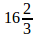%

b) 15%

c) 20%

d) 10%

e)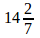Q.12) The average number of vehicles passing a bridge in a week is 1400. The average number of vehicles passing on Monday to Friday is 20% more than the average number of vehicles passing on Saturday and Sunday. What is the average number of vehicle passing the bridge on Monday to Friday?

a) 1750

b) 1470

c) 1400

d) 1635

e) 1530

Q.13) The ratio between the present age of a girl and her grandfather is 2 : 23, and the present age of the father of the girl is half of the age of her grandfather. The ratio between the present age of the mother and father of the girl is 19: 23, and the difference between the present age of the girl and her mother is 30. Then what is the total age of the girl and her father?

a) 52

b) 60

c) 40

d) 54

e) 38

D.14-17) Solve the equations and give the relationship between x and y.

Q.14)

I.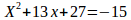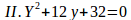a) X > Y

b) X < Y

c) X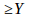d)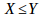e) X = Y (or) the relationship cannot be determined.

Q.15)

I.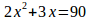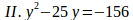a) X > Y

b) X < Y

c) Xd)e) X = Y (or) the relationship cannot be determined.

Q.16)

I. 13X + 14Y = 241

II. 9X + 5Y = 134

a) X > Y

b) X < Y

c) Xd)e) X = Y (or) the relationship cannot be determined.

Q.17)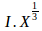+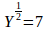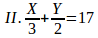a) X > Y

b) X < Y

c) Xd)e) X = Y (or) the relationship cannot be determined.

Q.18) The distance between point M and N is 720kms. The ratio of the speed of a cycle and motorcycle is 2 : 3. The cycle starts from point M, after 30 minutes the motorcycle starts from point N, what is the distance covered by cycle at the time of the meeting, if the two vehicles meet after 7.5hours from the cycle started.

a) 440kms

b) 300kms

c) 400kms

d) 420kms

e) 360kms

Q.19) The area of a rectangular room is equal to the area of a circular well. The diameter of the well is 14meters and the length is 3meters more than the breadth of the room, then what is the ratio between the perimeter of the room and circumference of the well?

a) 1 : 7

b) 25 : 22

c) 3 : 7

d) 5 : 9

e) 22 : 23

Q.20) A classroom contains some chairs and tables. The cost of the chair is 300 and the table is 1200, in what ratio both of them should be taken out from the class to get the average cost of Rs.800?

a) 3 : 4

b) 5 : 3

c) 4: 5

d) 2 : 3

e) 4 : 7

Q.1) c

Required difference =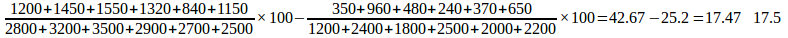Q.2) d

Required total = 480+840+1550+1740 + 370 + 740 + 840+ 2150 = 8710

Q.3) a

Required % =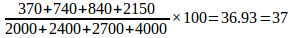Q.4) d

In 2014 =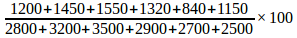= 42.67%

In 2013 =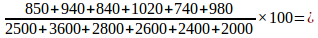33.77%

In 2012 =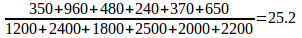In 2015 =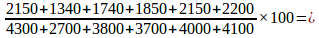50.57%

Q.5) c

Required %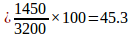Q.6) d

Required difference = 7510-3050= 4460

Q.7) b

Required total = 2800+ 3800 + 240+ 1850 = 8690

Q.8) b

Ratio of girls to boys in the school is 3: 2

From the difference, we can find the total number of students in the school = 3×300: 2×300= 900: 600

Number of students in the class =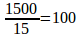Number of girls in the class =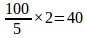Q.9) c

Sum =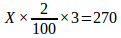Then Sum = Rs.4500.

Double the sum = Rs.9000,

Then the interest paid by him =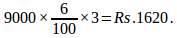Q.10) c

Profit ratio =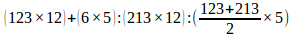= 1476+30 : 2556 : 168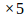= 1506 : 2556 : 840

= 251 : 426 : 140

Q.11) e

Selling price = Rs.100

Profit = 100 – 80 = 20

Discount = 20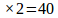Marked price = SP + Discount = 100 + 40 = 140

Then Required percentage =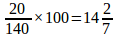%

Q.12) b

Weekend = 120% of 100

Weekend = 2 days

Week days = 5 days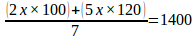Then X = 1225

120% of 1225 = 1470

Q.13) d

Age of the girl = 8

Grandfather = 92

Age of father = 46

Mother = 38

Then Father + girl = 46 + 8 = 54

Q.14) e

=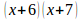Then X = -6, -7

= (Y + 4) (Y + 8)

Then Y = -4, -8

So, the relationship cannot be determined.

Q.15) b

=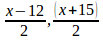X =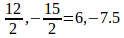=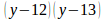Y = 12, 13

So, X < Y

Q.16) a

By solving the two equations we get, X = 11 and Y = 7

So, X > Y

Q.17) a

By solving the two equations, we get, the value of X = 27 and Y = 16

So, X > Y

Q.18) b

Cycle + motor cycle =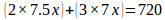=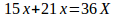Then, 36X = 720

Then, X = 20

Distance covered by cycle = 15X =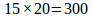kms

Q.19) b

The area of the well =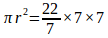= 154m2

Then, the length and breadth of room = 14 : 11

Perimeter of the room =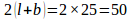m

Circumference of the well =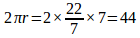m

Required ratio = 50 : 44 = 25 : 22

Q.20) c

Chair Table

300 1200

800

400 500

4 : 5

We will post this Practice Questions – Daily till the start of the IBPS RRB PO & Assistant (Regularly)

Quantitative Aptitude @ 3:00 P.M

Reasoning Ability -@ 5:00 P.M

Attend GEEK TEST – SOLVE 10 Daily and enhance your preparation to the next level. Attend the Daily Tests with the Timer and improve your solving ability.

Also Please attend this BLUE WHALE QUESTION (which is a combination of many topics and combined into a single one). This is a One Stop Solution for all your Miscellaneous needs.

## BLUE WHALE CHALLENGE QUESTIONS

Get the FULL LENGTH MOCK TEST SERIES for SBI PO Mains 2018 , SBI CLERK Mains 2018 & BOB PO Mains 2018 from our Bankersdaily Store.[social_warfare